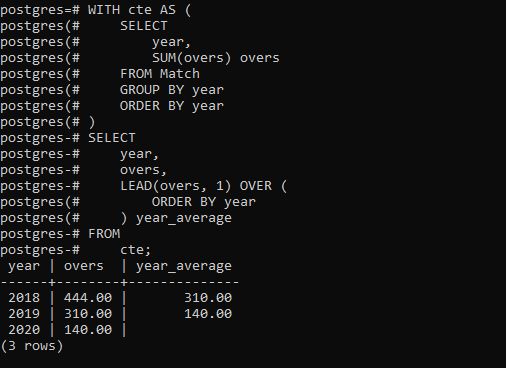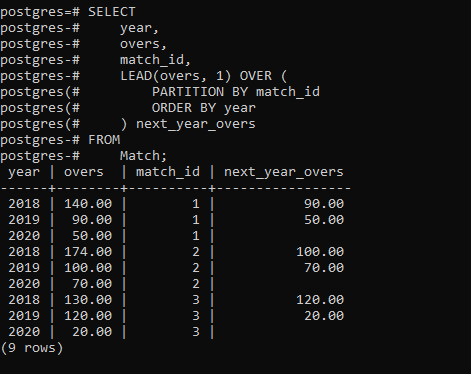• Last Updated : 10 Feb, 2021

In PostgreSQL, the LEAD() function is used to access a row that follows the current row, at a specific physical offset and is generally used for comparing the value of the current row with the value of the next row following the current row.

The syntax of LEAD() function looks like below:

```Syntax:
OVER (
[PARTITION BY partition_expression, ... ]
ORDER BY sort_expression [ASC | DESC], ...
)
```

Let’s analyze the above syntax:

• The expression can be a column, expression, subquery that must evaluate to a single value.
• The offset is a positive integer that specifies the number of rows forwarding from the current row. It is generally an expression, subquery, or column. If offset is not set, it defaults to 1.
• The PARTITION BY clause divides rows into partitions. By default, it takes the query result as a single partition.
• The ORDER BY clause is used to sort the query result rows in each partition.

Example 1:

Let’s set up a new table for the demonstration named Match:

```CREATE TABLE Match(
year SMALLINT CHECK(year > 0),
match_id INT NOT NULL,
overs DECIMAL(10,2) NOT NULL,
PRIMARY KEY(year,match_id)
);
```

Now insert some data to it:

```INSERT INTO
Match(year, match_id, overs)
VALUES
(2018, 1, 140),
(2018, 2, 174),
(2018, 3, 130),
(2019, 1, 90),
(2019, 2, 100),
(2019, 3, 120),
(2020, 1, 50),
(2020, 2, 70),
(2020, 3, 20);
```

The below query uses the LEAD() function to return the overs of the current year and the average overs per year:

```WITH cte AS (
SELECT
year,
SUM(overs) overs
FROM Match
GROUP BY year
ORDER BY year
)
SELECT
year,
overs,
ORDER BY year
) year_average
FROM
cte;
```

Output:Example 2:

The following statement uses the LEAD() function to compare the overs of the current year with overs of the next year for each group:

```SELECT
year,
overs,
match_id,
PARTITION BY match_id
ORDER BY year
) next_year_overs
FROM
Match;
```

Output:My Personal Notes arrow_drop_up